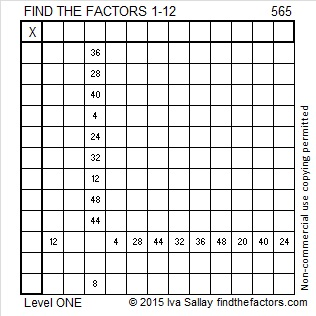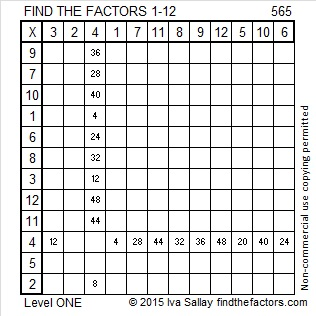# 565 and Level 1

565 is the sum of consecutive primes: 181 + 191 + 193 = 565.

565 is the sum of two squares two different ways: 565 = (22^2) + (9^2), and 565 = (23^2) + (6^2).

565 is the hypotenuse of four Pythagorean triples. The greatest common factor of two of them is 1 because they are primitives. Which of these triples are not primitive, and what is the greatest common factor of each of them?

• 75-560-565
• 276-493-565
• 339-452-565
• 396-403-565Print the puzzles or type the solution on this excel file: 12 Factors 2015-07-27

—————————————————————————————————

• 565 is a composite number.
• Prime factorization: 565 = 5 x 113
• The exponents in the prime factorization are 1 and 1. Adding one to each and multiplying we get (1 + 1)(1 + 1) = 2 x 2 = 4. Therefore 565 has exactly 4 factors.
• Factors of 565: 1, 5, 113, 565
• Factor pairs: 565 = 1 x 565 or 5 x 113
• 565 has no square factors that allow its square root to be simplified. √565 ≈ 23.7697—————————————————————————————————This site uses Akismet to reduce spam. Learn how your comment data is processed.